# Linear basis function models with PyMC4¶

In :
import logging
import pymc4 as pm
import numpy as np
import arviz as az

import tensorflow as tf
import tensorflow_probability as tfp

print(pm.__version__)
print(tf.__version__)
print(tfp.__version__)

# Mute Tensorflow warnings ...
logging.getLogger('tensorflow').setLevel(logging.ERROR)

4.0a2
2.2.0-dev20200414
0.10.0-dev20200414


## Linear basis function models¶

The following is a PyMC4 implementation of Bayesian regression with linear basis function models. To recap, a linear regression model is a linear function of the parameters but not necessarily of the input. Input $x$ can be expanded with a set of non-linear basis functions $\phi_j(x)$, where $(\phi_1(x), \dots, \phi_M(x))^T = \boldsymbol\phi(x)$, for modeling a non-linear relationship between input $x$ and a function value $y$.

$$y(x, \mathbf{w}) = w_0 + \sum_{j=1}^{M}{w_j \phi_j(x)} = w_0 + \mathbf{w}_{1:}^T \boldsymbol\phi(x) \tag{1}$$

For simplicity I'm using a scalar input $x$ here. Target variable $t$ is given by the deterministic function $y(x, \mathbf{w})$ and Gaussian noise $\epsilon$.

$$t = y(x, \mathbf{w}) + \epsilon \tag{2}$$

Here, we can choose between polynomial and Gaussian basis functions for expanding input $x$.

In :
from functools import partial
from scipy.stats import norm

def polynomial_basis(x, power):
return x ** power

def gaussian_basis(x, mu, sigma):
return norm(loc=mu, scale=sigma).pdf(x).astype(np.float32)

def _expand(x, bf, bf_args):
return np.stack([bf(x, bf_arg) for bf_arg in bf_args], axis=1)

def expand_polynomial(x, degree=3):
return _expand(x, bf=polynomial_basis, bf_args=range(1, degree + 1))

def expand_gaussian(x, mus=np.linspace(0, 1, 9), sigma=0.3):
return _expand(x, bf=partial(gaussian_basis, sigma=sigma), bf_args=mus)

# Choose between polynomial and Gaussian expansion
# (by switching the comment on the following two lines)
expand = expand_polynomial
#expand = expand_gaussian


For example, to expand two input values [0.5, 1.5] into a polynomial design matrix of degree 3 we can use

In :
expand_polynomial(np.array([0.5, 1.5]), degree=3)

Out:
array([[0.5  , 0.25 , 0.125],
[1.5  , 2.25 , 3.375]])

The power of 0 is omitted here and covered by a $w_0$ in the model.

## Example dataset¶

The example dataset consists of N noisy samples from a sinusoidal function f.

In :
import matplotlib.pyplot as plt
%matplotlib inline

from bayesian_linear_regression_util import (
plot_data,
plot_truth
)

def f(x, noise=0):
"""Sinusoidal function with optional Gaussian noise."""
return 0.5 + np.sin(2 * np.pi * x) + np.random.normal(scale=noise, size=x.shape)

# Number of samples
N = 10

# Constant noise
noise = 0.3

# Noisy samples
x = np.linspace(0, 1, N, dtype=np.float32)
t = f(x, noise=noise)

# Noise-free ground truth
x_test = np.linspace(0, 1, 100).astype(np.float32)
y_true = f(x_test)

plot_data(x, t)
plot_truth(x_test, y_true)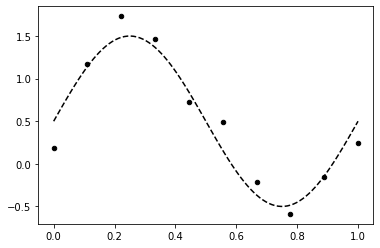## Implementation with PyMC4¶

### Model definition¶

The model definition directly follows from Eq. $(1)$ and Eq. $(2)$ with normal priors over parameters. The size of parameter vector w_r ($\mathbf{w}_{1:}$ in Eq. $(1)$) is determined by the number of basis functions and set via the batch_stack parameter. With the above default settings, it is 3 for polynomial expansion and 9 for Gaussian expansion.

In :
import tensorflow as tf

@pm.model
def model(Phi, t, sigma=noise):
"""Linear model generator.

Args:
- Phi: design matrix (N,M)
- t: noisy target values (N,)
- sigma: known noise of t
"""

w_0 = yield pm.Normal(name='w_0', loc=0, scale=10)
w_r = yield pm.Normal(name='w_r', loc=0, scale=10, batch_stack=Phi.shape)

mu = w_0 + tf.tensordot(w_r, Phi.T, axes=1)

yield pm.Normal(name='t_obs', loc=mu, scale=sigma, observed=t)


### Inference¶

Tensorflow will automatically run inference on a GPU if available. With the current version of PyMC4, inference on a GPU is quite slow compared to a multi-core CPU (need to investigate that in more detail). To enforce inference on a CPU set environment variable CUDA_VISIBLE_DEVICES to an empty value. There is no progress bar visible yet during sampling but the following shouldn't take longer than a 1 minute.

In :
trace = pm.sample(model(expand(x), t), num_chains=3, burn_in=100, num_samples=1000)

In :
az.plot_trace(trace);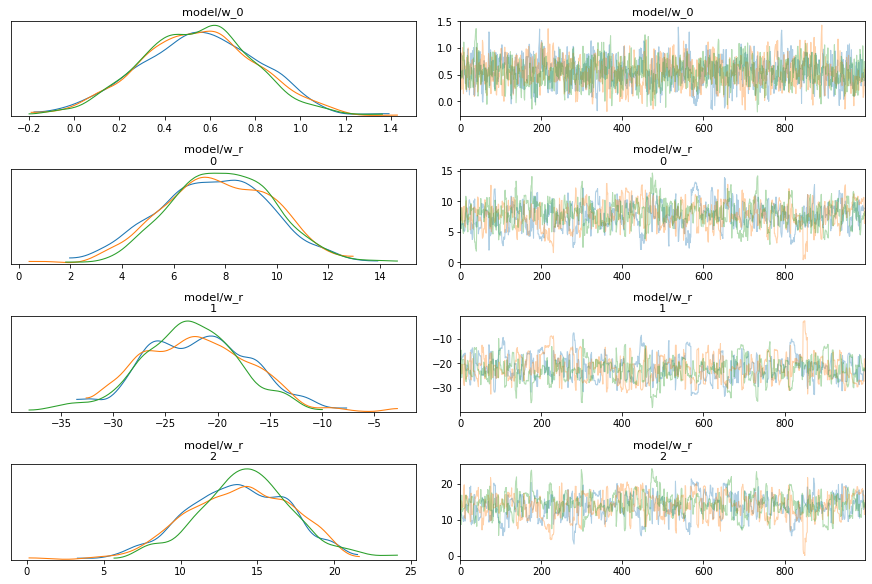In :
az.plot_posterior(trace, var_names="model/w_0");
az.plot_posterior(trace, var_names="model/w_r");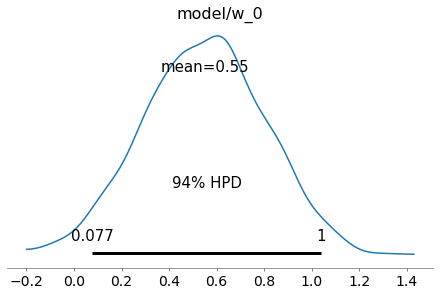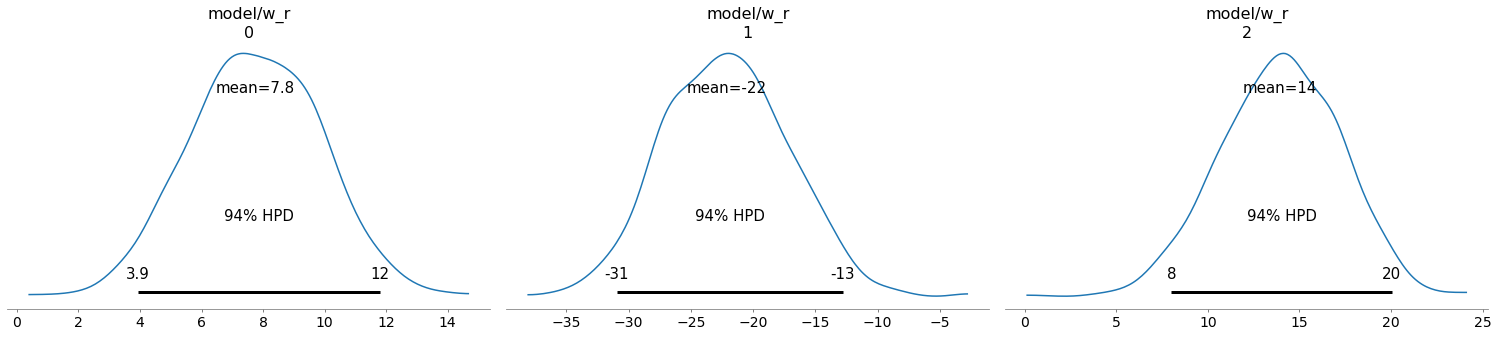### Prediction¶

To obtain posterior predictive samples for a test set x_test we simply call the model generator function again with the expanded test set. This is a nice improvement over PyMC3 which required to setup a shared Theano variable for setting test set values. Target values are ignored during predictive sampling, only the shape of the target array t matters.

In :
draws_posterior = pm.sample_posterior_predictive(model(expand(x_test), t=np.zeros_like(x_test)), trace, inplace=False)
draws_posterior.posterior_predictive

Out:
xarray.Dataset
• chain: 3
• draw: 1000
• model/t_obs_dim_0: 100
• chain
(chain)
int64
0 1 2
array([0, 1, 2])
• draw
(draw)
int64
0 1 2 3 4 5 ... 995 996 997 998 999
array([  0,   1,   2, ..., 997, 998, 999])
• model/t_obs_dim_0
(model/t_obs_dim_0)
int64
0 1 2 3 4 5 6 ... 94 95 96 97 98 99
array([ 0,  1,  2,  3,  4,  5,  6,  7,  8,  9, 10, 11, 12, 13, 14, 15, 16, 17,
18, 19, 20, 21, 22, 23, 24, 25, 26, 27, 28, 29, 30, 31, 32, 33, 34, 35,
36, 37, 38, 39, 40, 41, 42, 43, 44, 45, 46, 47, 48, 49, 50, 51, 52, 53,
54, 55, 56, 57, 58, 59, 60, 61, 62, 63, 64, 65, 66, 67, 68, 69, 70, 71,
72, 73, 74, 75, 76, 77, 78, 79, 80, 81, 82, 83, 84, 85, 86, 87, 88, 89,
90, 91, 92, 93, 94, 95, 96, 97, 98, 99])
• model/t_obs
(chain, draw, model/t_obs_dim_0)
float32
0.62803966 ... -0.10609433
array([[[ 6.2803966e-01,  3.0982676e-01,  1.3288246e+00, ...,
2.0092756e-02, -4.6279129e-01, -3.5547027e-01],
[ 1.2540956e+00,  1.3001926e+00,  4.7648013e-01, ...,
-1.4047767e-01, -6.6063479e-02,  2.2666046e-01],
[ 8.7482959e-01,  7.1901262e-01,  1.2609010e+00, ...,
3.6891103e-01,  2.3930666e-01,  1.9714403e-01],
...,
[ 6.5140450e-01,  9.2145377e-01,  2.7004269e-01, ...,
6.3866097e-04,  3.6582848e-01,  4.0039763e-01],
[ 4.4500881e-01,  2.6833433e-01,  5.4804039e-01, ...,
7.7021873e-01,  2.5889888e-02,  6.1815977e-03],
[ 7.7372921e-01,  7.9454470e-01,  6.1503142e-01, ...,
1.0394448e-01, -4.7731856e-01, -6.0296464e-01]],

[[ 1.0802209e-02,  5.3853476e-01,  4.2005211e-01, ...,
3.4785268e-01,  5.5825341e-01,  3.6537340e-01],
[ 1.0661882e+00,  6.1011136e-01,  1.2609197e+00, ...,
2.7852780e-01,  6.0179305e-01,  8.8738966e-01],
[ 7.4540353e-01,  1.2344036e+00,  1.2811742e+00, ...,
7.6069474e-01,  5.6832170e-01,  1.1162102e+00],
...,
[-9.9507570e-03,  3.3239186e-01,  4.7235852e-01, ...,
-3.1367943e-01, -1.1621615e-01,  8.4965013e-02],
[ 6.0881937e-01,  5.4845160e-01,  3.3895850e-01, ...,
-1.8985049e-01,  5.1551007e-02, -2.9580078e-01],
[ 4.2067486e-01,  1.0590549e+00,  8.4452099e-01, ...,
-4.9186319e-01, -1.4563501e-01, -1.7367038e-01]],

[[-2.7087212e-01,  2.2036786e-01,  2.1426165e-01, ...,
1.7241767e-01,  3.1225359e-01,  6.8863893e-01],
[ 7.2146583e-01,  6.2246352e-01,  6.6259170e-01, ...,
3.3384523e-01, -2.5926927e-01, -3.3233041e-01],
[ 4.1656739e-01,  7.5270784e-01,  5.5890125e-01, ...,
1.1958110e-01, -1.2425715e-01, -2.5198370e-01],
...,
[ 5.0131804e-01, -7.0139110e-02,  1.1371263e+00, ...,
1.8864080e-01, -1.3917631e-01,  4.4616908e-01],
[ 1.9719602e-01,  6.5913051e-01,  8.3163023e-01, ...,
5.0224549e-01,  4.1368300e-01,  5.7770413e-01],
[ 5.0372481e-01,  3.2341504e-01,  6.1320949e-01, ...,
-6.8751842e-02, -7.4040598e-01, -1.0609433e-01]]], dtype=float32)
• created_at :
2020-04-22T05:50:53.606431
arviz_version :
0.7.0

The predictive mean and standard deviation is obtained by averaging over chains (axis 0) and predictive samples (axis 1) for each of the 100 data points in x_test (axis 2).

In :
predictive_samples = draws_posterior.posterior_predictive.data_vars['model/t_obs'].values

m = np.mean(predictive_samples, axis=(0, 1))
s = np.std(predictive_samples, axis=(0, 1))


These statistics can be used to plot model predictions and their uncertainties (together with the ground truth and the noisy training dataset).

In :
plt.fill_between(x_test, m + s, m - s, alpha = 0.5, label='Predictive std. dev.')
plt.plot(x_test, m, label='Predictive mean');

plot_data(x, t)
plot_truth(x_test, y_true, label=None)

plt.legend();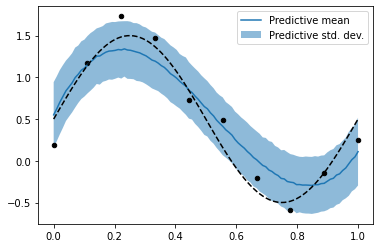Try running the example again with Gaussian expansion i.e. setting expand = expand_gaussian and see how it compares to polynomial expansion. Also try running with a different number of basis functions by overriding the default arguments of expand_polynomial and expand_gaussian. You can find more PyMC4 examples in the notebooks diretory of the PyMC4 project.